# Problem 9.5 Part A At t 5 ms, a sinusoidal current is known to be zero...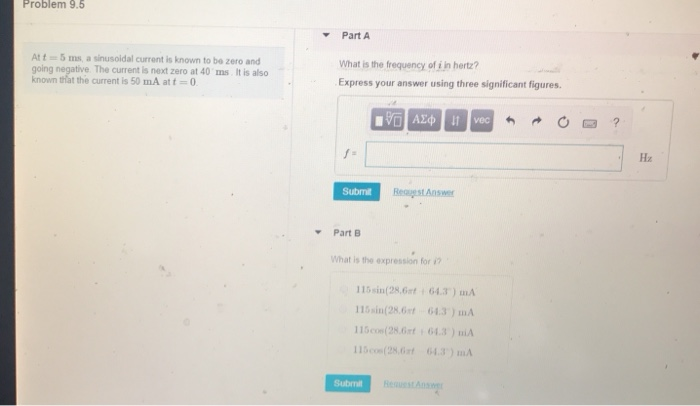Problem 9.5 Part A At t 5 ms, a sinusoidal current is known to be zero and going negative. The current is next zero at 40 ms. It is also known tiat the current is 50 mA at t-0 What is the frequency of i in hertz? Express your answer using three significant figures Submit Reauest Answer Part B What is the expression for 115sin(28,Gat 64.3) mA 15sin(28.6t 64.3) mA 115cos(28.6rt:64.3) niA 15cos(28.6t 643) mA Submi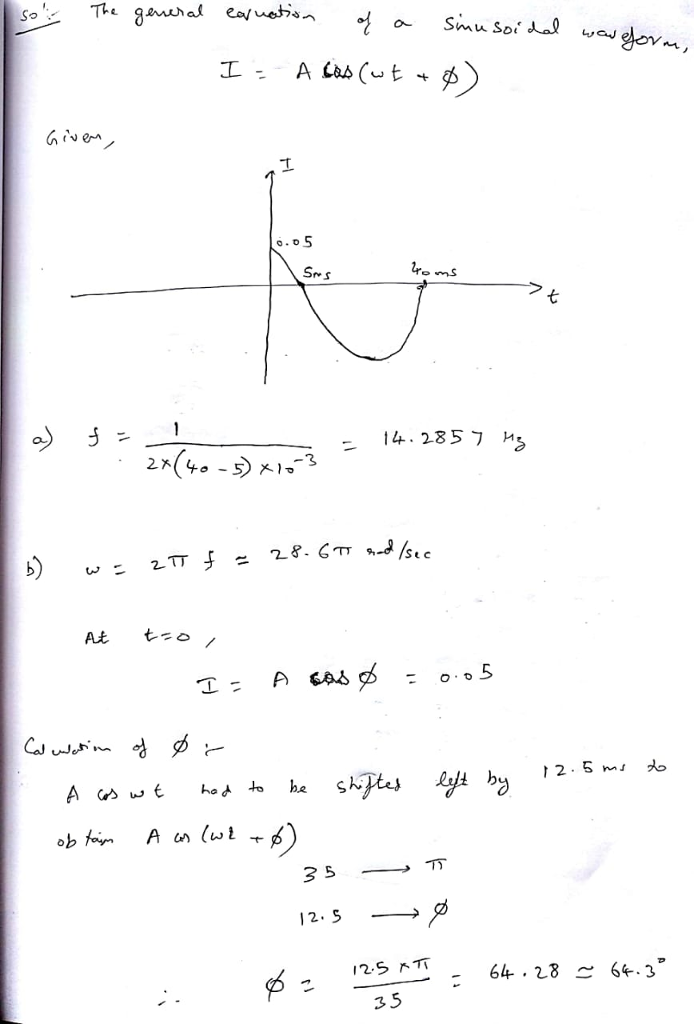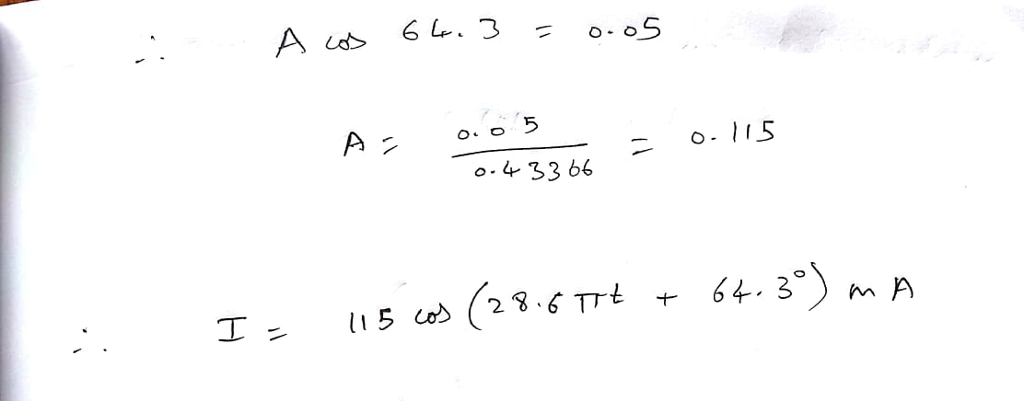#### Earn Coin

Coins can be redeemed for fabulous gifts.

Similar Homework Help Questions
• ### Problem 9.13 Review 1 Constants Part A A 380 Hz sinusoidal voltage with a maximum amplitude...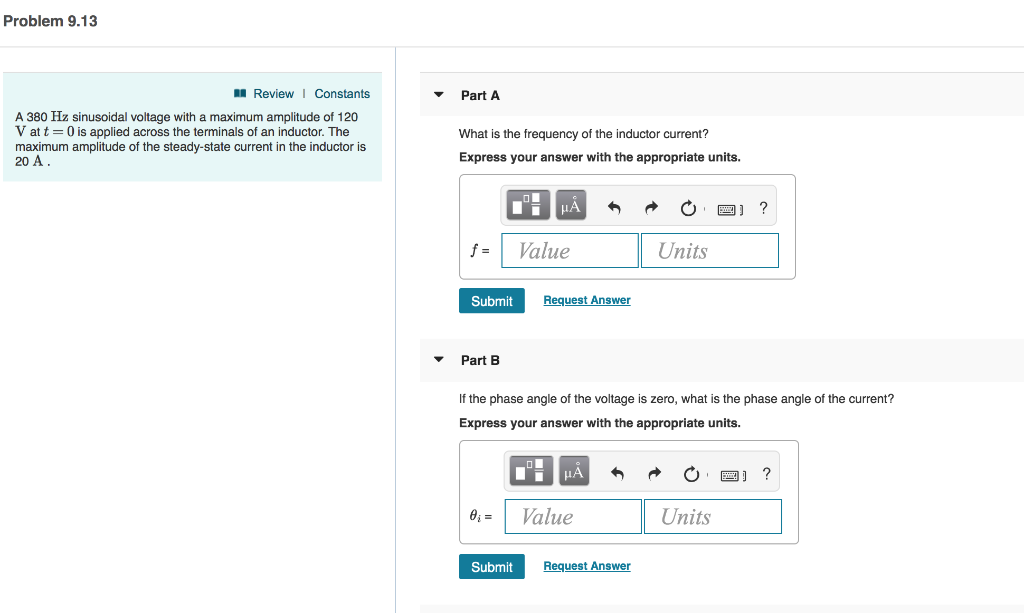Problem 9.13 Review 1 Constants Part A A 380 Hz sinusoidal voltage with a maximum amplitude of 120 V at t 0 is applied across the terminals of an inductor. The maximum amplitude of the steady-state current in the inductor is 20 A What is the frequency of the inductor current? Express your answer with the appropriate units. fValue Units Submit Request Answer Part B If the phase angle of the voltage is zero, what is the phase angle of...

• ### Problem 6.1 PSpice Multisim PartA The current in a 150 H inductor is known to be...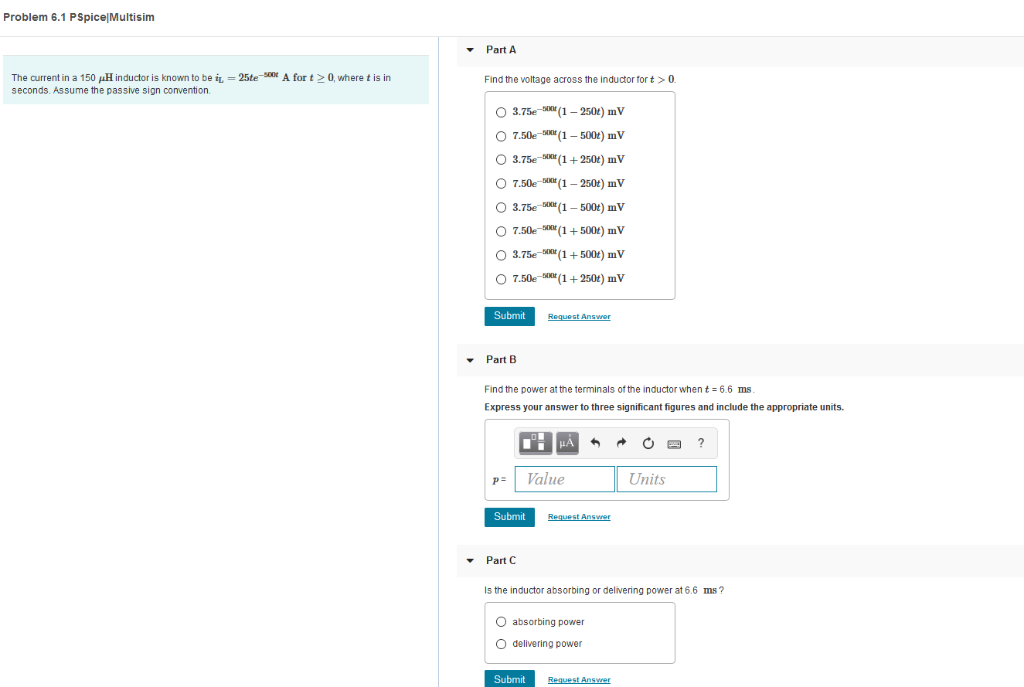Problem 6.1 PSpice Multisim PartA The current in a 150 H inductor is known to be i seconds. Assume the passive sign convention 25teA for t0, where t is in Find the voltage across the inductor fort>0 O 7.50e (1 500t mV O 3.75e 250t) mV O 7.50e-50"(1-250t) mV O 3.75e SKM (1- 500t) mV O 7.50e-00t) mV 3.75e(+500) mV O 7.50e 250t) mV Submit PartB Find the power at the terminals of the inductor when t 6.6 ms Express...

• ### PLEASE BOX THE ANSWERS FOR A THUMBS UP Problem 6.9 The current in a 15 mH...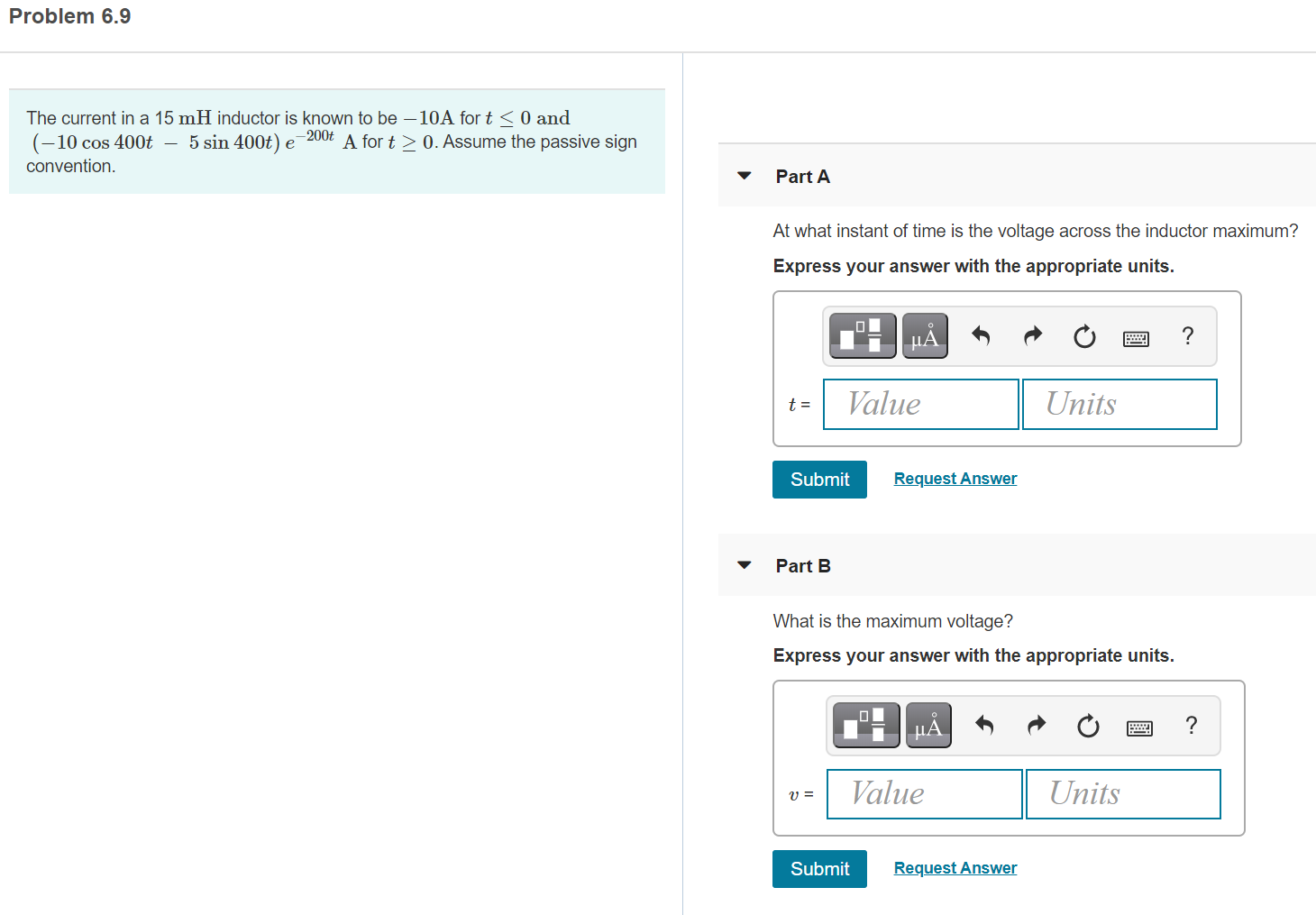PLEASE BOX THE ANSWERS FOR A THUMBS UP Problem 6.9 The current in a 15 mH inductor is known to be -10A fort < 0 and (-10 cos 400t – 5 sin 400t) e -2004 A fort > 0. Assume the passive sign convention. Part A At what instant of time is the voltage across the inductor maximum? Express your answer with the appropriate units. μΑ ? t = Value Units Submit Request Answer Part B What is the maximum...

• ### The current in a 200 mH inductor is i = 75 mA, t < 0; -50+...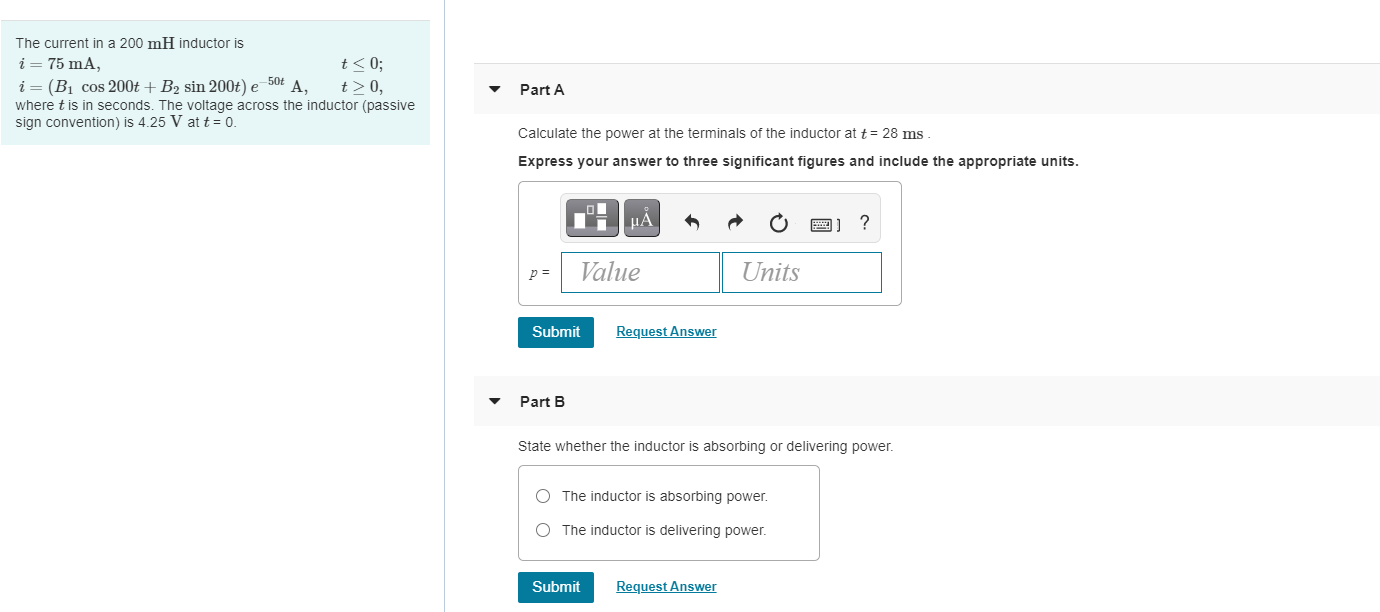The current in a 200 mH inductor is i = 75 mA, t < 0; -50+ i= (B1 cos 200t + B2 sin 200t) e A, t>0, where t is in seconds. The voltage across the inductor (passive sign convention) is 4.25 V at t = 0. Part A Calculate the power at the terminals of the inductor at t = 28 ms Express your answer to three significant figures and include the appropriate units. μΑ p = Value Units...

• ### Problem 1.20 The voltage and current at the terminals of the circuit element in (Figure 1)...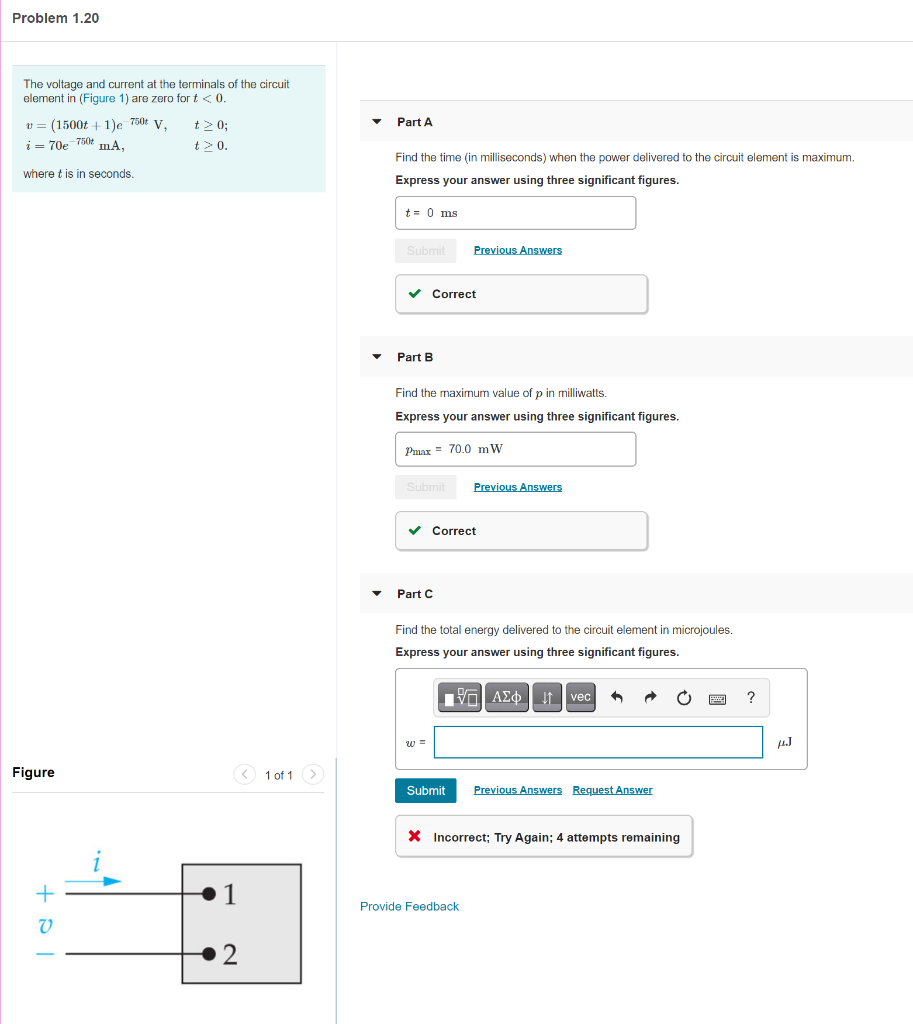Problem 1.20 The voltage and current at the terminals of the circuit element in (Figure 1) are zero fort < 0. u= (1500t + 1)e 750t V t> 0; i= 70e 7500 mA, t> 0. Part A where t is in seconds Find the time (in milliseconds) when the power delivered to the circuit element is maximum. Express your answer using three significant figures. t = 0 ms Submit Previous Answers Correct Part B Find the maximum value of p...

• ### Problem 5 (20 Points): For the circuit shown below, the input is the current source, I(t) and the...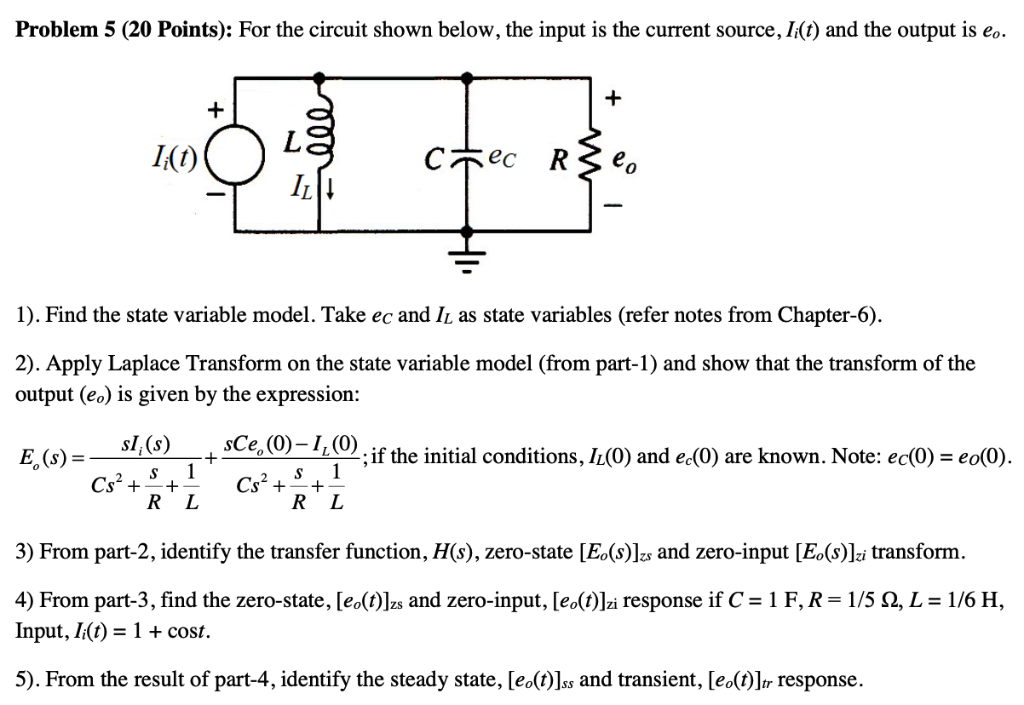Problem 5 (20 Points): For the circuit shown below, the input is the current source, I(t) and the output is eo. 1). Find the state variable model. Take ec and IL as state variables (refer notes from Chapter-6). 2). Apply Laplace Transform on the state variable model (from part-1) and show that the transform of the output (eo) is given by the expression: 사스 ; if the initial conditions, L(0) and ec(0) are known. Note: ec(0)-eo(0) R L R L...

• ### Item 1 1 of 1 Part A Constants What is the values of the constant A in the followin equetion? An L-R-C series circuit has L = 0.450 H. C 6.00 F, and R 380 2. At t 0 the current is zero and the initia...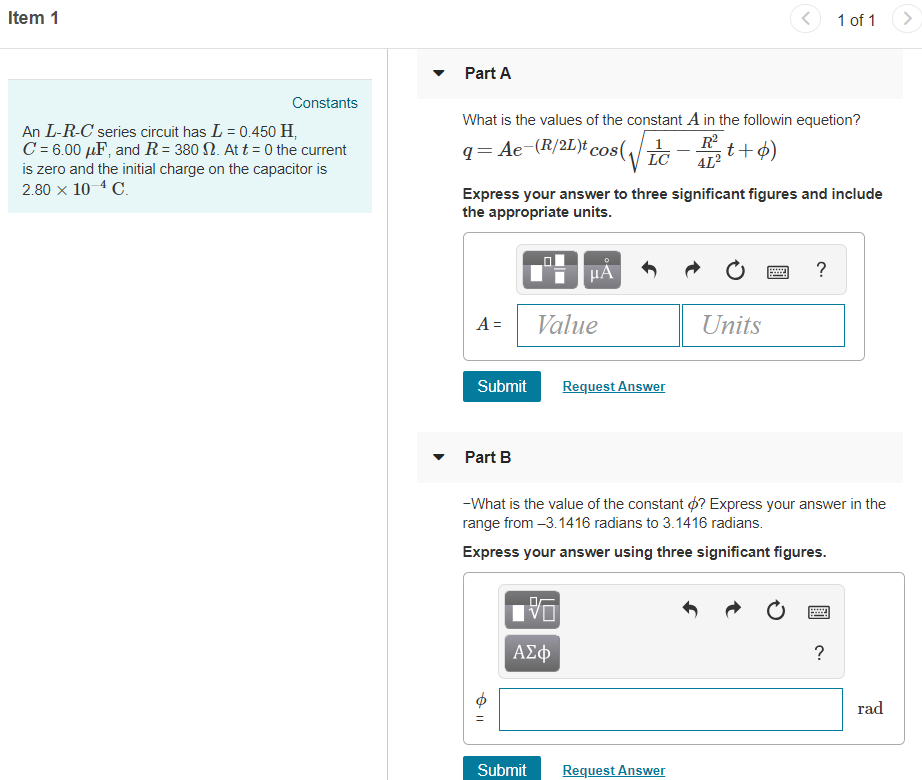Item 1 1 of 1 Part A Constants What is the values of the constant A in the followin equetion? An L-R-C series circuit has L = 0.450 H. C 6.00 F, and R 380 2. At t 0 the current is zero and the initial charge on the capacitor is 2.80× 10 4 C Express your answer to three significant figures and include the appropriate units AValue Units Submit Request Answer Part B -What is the value of the...

• ### Please help! and do not use rounding until the very end. answer must be very accurate. Item 1 Canstants Part A AnE-R-C senes circut has L=0450 HC=700 /F. and R=300 Ω At t=¢the current 18 zero and the...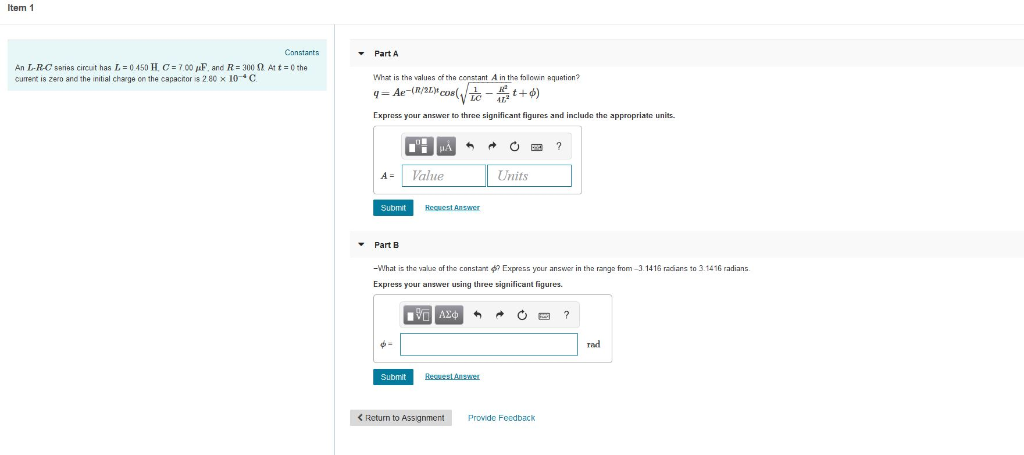Please help! and do not use rounding until the very end. answer must be very accurate. Item 1 Canstants Part A AnE-R-C senes circut has L=0450 HC=700 /F. and R=300 Ω At t=¢the current 18 zero and the initial charge on the capacnora 280 10-4 C What is tha valuas of tha constant A in tha follewin aquation? Express your answer to three significant figures and include the appropriate units A-1 Value Units Submit Part B -What is the value...

• ### Problem 6.16 7 of 23 Review Constants An uncharged 0.2 μ capacitor is driven by a triangular current pulse. The current pulse is described by: t0s; O, 5000t A 10.2-50001 A, The vollage is described b...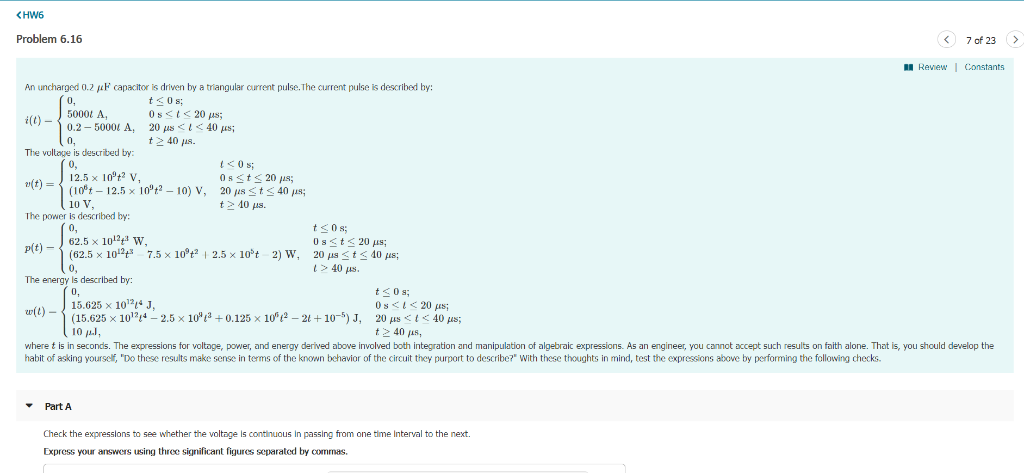Problem 6.16 7 of 23 Review Constants An uncharged 0.2 μ capacitor is driven by a triangular current pulse. The current pulse is described by: t0s; O, 5000t A 10.2-50001 A, The vollage is described by: r(t) = 12.5 × 10,2 v 20 μ.<1<40pcs; t40 ps. 0, 0 s; 0st 20 us; 20 MS t-40 μ8; t40 s. - (loft-12.5 x 10":2-10) V, 10 V The power is described by: tS0s 0. p(t)-62.5 x 1012tt w 1>, 40μ. 0. The...